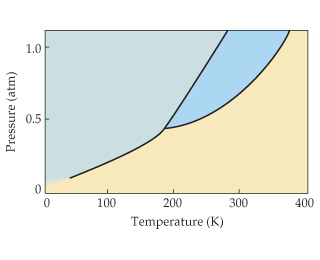# Problem: The phase diagram of a hypothetical substance is shown below.Estimate the normal boiling point of the substance.

###### FREE Expert Solution

We’re being asked to determine the normal boiling point of a given substance base on its phase diagram

Recall that a phase diagram shows the transition of matter between solid, liquid, and gas phases as temperature and pressure changes.

In a phase diagram, the different phases can be identified by their location:

• Solid: can be found at high pressure and low temperature; labeled A in the phase diagram

• Liquid: can be found between the solid and gas regions; labeled B

• Gas: can be found at low pressure and high temperature; labeled C

90% (121 ratings)###### Problem Details

The phase diagram of a hypothetical substance is shown below.Estimate the normal boiling point of the substance.